# How to obtain the daily interest amount (per diem) in Margill Loan Manager

Q: How to obtain the daily interest amount (per diem) in Margill Loan Manager?

A: This can be added very easily through a simple Mathematical Equation.

Go to Reports > Equation Management and click on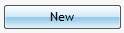1. Name the Equation
2. Select the two required fields (balance and interest rate) from the various themes on the left
3. Add the operators with the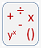button
4. Divide by 365 days with the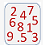button
5. Save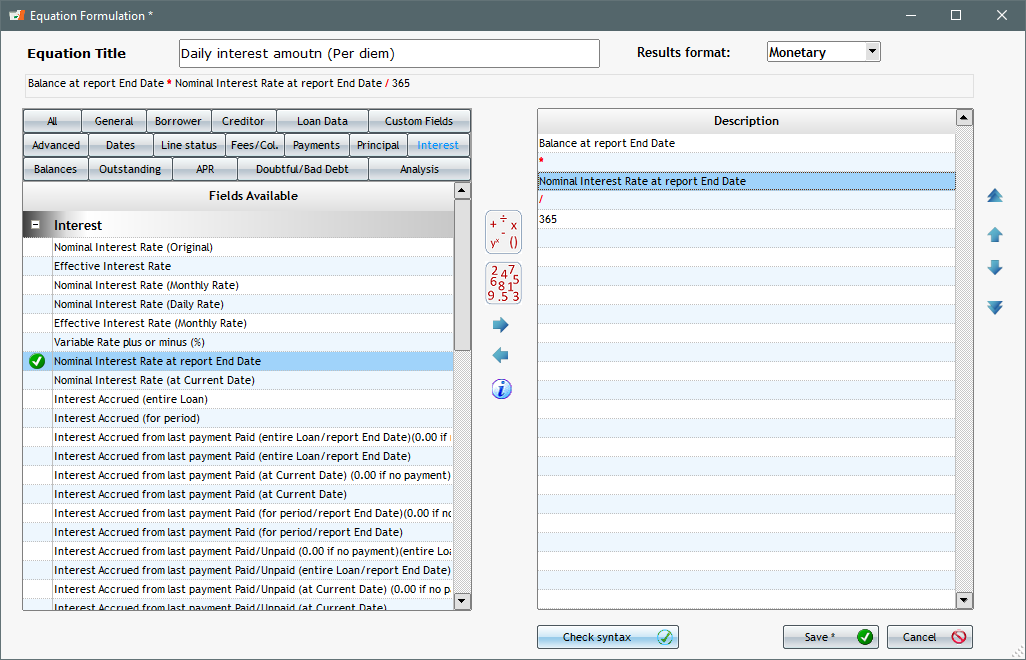You can now use this simple interest Equation in various reports picking it up in the Equations theme:

Record List Customized: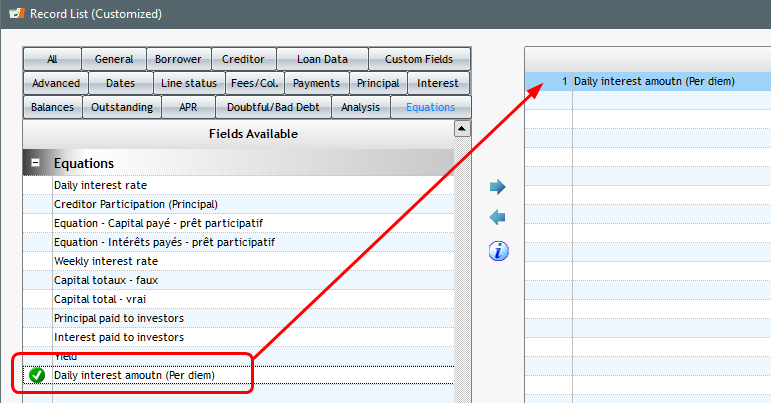Document Merge (DOCX., RTF, PDF) for your Invoices and Statements: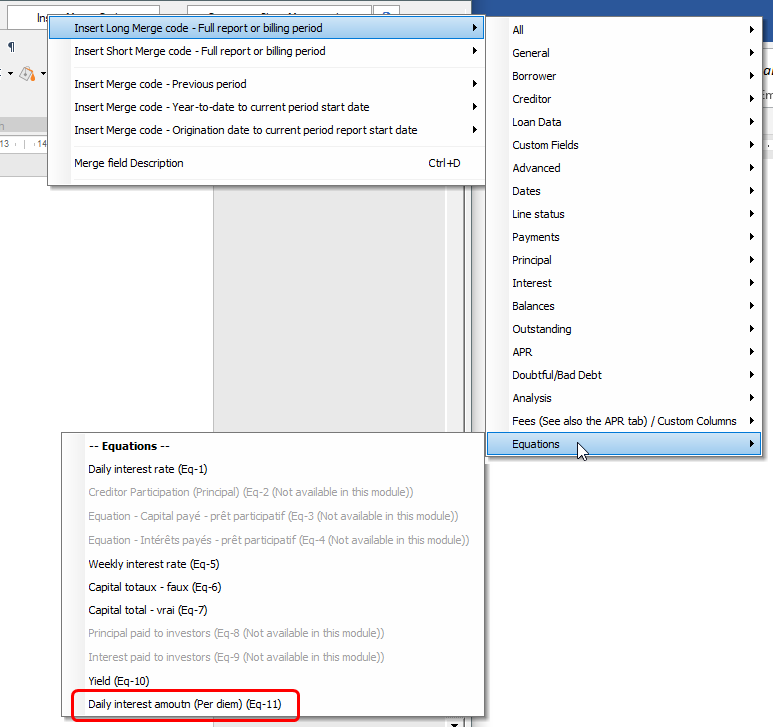Tags: